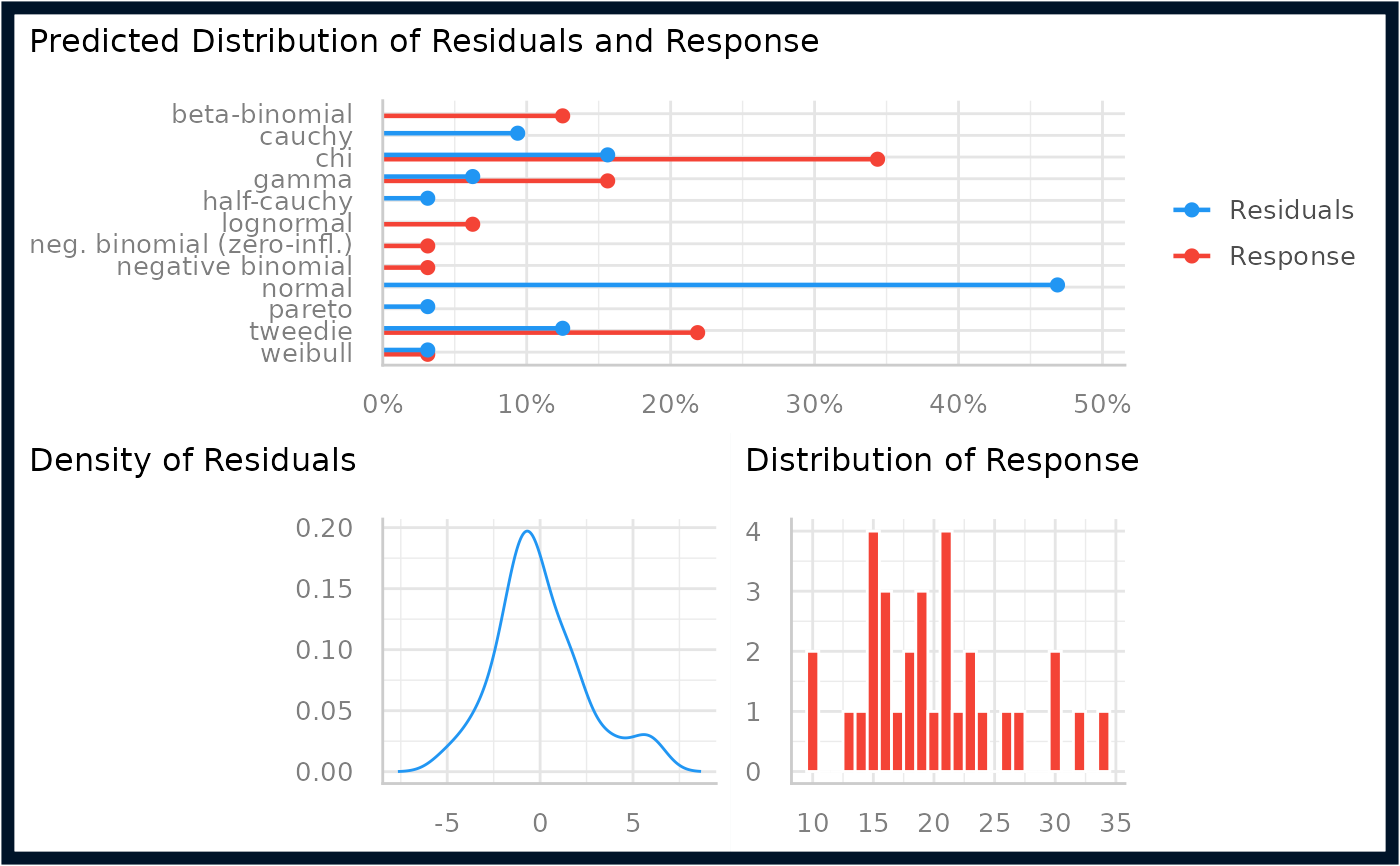The plot() method for the performance::check_distribution() function.

## Usage

# S3 method for see_check_distribution
plot(x, size_point = 2, panel = TRUE, ...)

## Arguments

x

An object.

size_point

Numeric specifying size of point-geoms.

panel

Logical, if TRUE, plots are arranged as panels; else, single plots are returned.

...

Arguments passed to or from other methods.

## Value

A ggplot2-object.

## Examples

library(performance)
m <<- lm(mpg ~ wt + cyl + gear + disp, data = mtcars)
result <- check_distribution(m)
result
#> # Distribution of Model Family
#>
#> Predicted Distribution of Residuals
#>
#>  Distribution Probability
#>        normal         47%
#>        cauchy         34%
#>       tweedie          6%
#>
#> Predicted Distribution of Response
#>
#>   Distribution Probability
#>        tweedie         44%
#>            chi         28%
#>  beta-binomial         16%
plot(result)